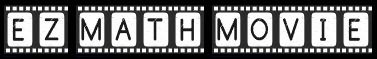# Linear Functions and Equations, General Form

### 0 = Ax + By + C

The formula 0 = Ax + By + C is said to be the 'general form' for the equation of a line. A, B, and C are three real numbers. Once these are given, the values for x and y that make the statement true express a set, or locus, of (x, y) points which form a certain line.

### Changing to slope-intercept form

At first it is difficult to imagine the slant and position of the line just by looking at the general form. However, we can change the general form into slope-intercept form and then get a good idea of what we are looking at. Here's the algebra:

 0 = Ax + By + C Starting equation 0 - By = Ax + By -By + C Subtract By from each side -By = Ax + C Left side: 0 - By = -ByRight side: By - By = 0 -By/-B = Ax/-B + C/-B Divide each side by -B y = (-A/B)x + (-C/B) Left side: -By/-B = yRight side: Ax/-B = (-A/B)xRight side: C/-B = (-C/B) y = mx + b Where: m = (-A/B)Where: b = (-C/B)

### Again, where are the slope and y-intercept?

As the previous algebra has shown, if we start with this general form:

0 = Ax + By + C

Then the slope of the line is:

slope = m = -A/B

And the y-intercept is:

y-intercept = b = -C/B

### A simple example

0 = Ax + By + C

Let:

A = 2, B = 3, C = 4

So:

0 = 2x + 3y + 4

slope = m = -A/B = -2/3 = -0.67 (approximately)

y-intercept = b = -C/B = -4/3 = -1.33 (approximately)

Our line in slope-intercept form:

y = mx + b

y = -0.67x + (-1.33) or y = -0.67x - 1.33

### The following interactive application

Below is a graph that presents a line as it is given by the general form equation. You can control the example values for A, B, and C by clicking their appropriate '+' and '-' buttons. The current general form equation is shown in the upper left corner of the graph.

This is an EZ Math Movie. Click the 'Show system' checkbox to expose the EZ Math Movie language. You do not need to understand EZ Math Movie to use this application.

### General form for the equation of a line: 0 = Ax + By + C

0ptions

Go on input

Bounds:
Sorry, apparently this browser does not support HTML 5 canvas tag graphics.
Printer

Edit: Runtime          View: Starter Suggestion

To runtime:

Storage:

Editor width: Normal Wide

### Original page

The original page for this information contains a Java applet animation that resembles the application found here. If your browser runs Java, you may want to take a look at it. You can find it by clicking here.

Custom SearchThis page contains an EZ Math Movie application. EZ Math Movie is an animation programming language that helps you experiment with mathematics. Learn more about it at ezmathmovie.com

Name:
Domain:

Name
0

Name:
Name
0